# Resources tagged with: Polyhedra

Filter by: Content type:
Age range:
Challenge level:

### There are 22 results

Broad Topics > 3D Geometry, Shape and Space > Polyhedra### Lighting up Time

##### Age 7 to 14 Challenge Level:

A very mathematical light - what can you see?### Dodecawhat

##### Age 14 to 16 Challenge Level:

Follow instructions to fold sheets of A4 paper into pentagons and assemble them to form a dodecahedron. Calculate the error in the angle of the not perfectly regular pentagons you make.### Three Cubes

##### Age 14 to 16 Challenge Level:

Can you work out the dimensions of the three cubes?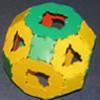### Platonic and Archimedean Solids

##### Age 7 to 16 Challenge Level:

In a recent workshop, students made these solids. Can you think of reasons why I might have grouped the solids in the way I have before taking the pictures?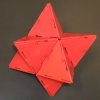### Investigating Solids with Face-transitivity

##### Age 14 to 18

In this article, we look at solids constructed using symmetries of their faces.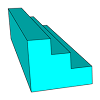### Which Solid?

##### Age 7 to 16 Challenge Level:

This task develops spatial reasoning skills. By framing and asking questions a member of the team has to find out what mathematical object they have chosen.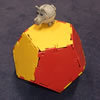### Platonic Planet

##### Age 14 to 16 Challenge Level:

Glarsynost lives on a planet whose shape is that of a perfect regular dodecahedron. Can you describe the shortest journey she can make to ensure that she will see every part of the planet?### Sliced

##### Age 14 to 16 Challenge Level:

An irregular tetrahedron has two opposite sides the same length a and the line joining their midpoints is perpendicular to these two edges and is of length b. What is the volume of the tetrahedron?### Tetra Square

##### Age 11 to 14 Challenge Level:

ABCD is a regular tetrahedron and the points P, Q, R and S are the midpoints of the edges AB, BD, CD and CA. Prove that PQRS is a square.### Tet-trouble

##### Age 14 to 16 Challenge Level:

Is it possible to have a tetrahedron whose six edges have lengths 10, 20, 30, 40, 50 and 60 units?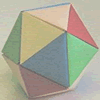### Paper Folding - Models of the Platonic Solids

##### Age 11 to 16

A description of how to make the five Platonic solids out of paper.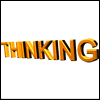### Thinking 3D

##### Age 7 to 14

How can we as teachers begin to introduce 3D ideas to young children? Where do they start? How can we lay the foundations for a later enthusiasm for working in three dimensions?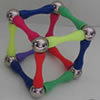### Magnetic Personality

##### Age 7 to 16 Challenge Level:

60 pieces and a challenge. What can you make and how many of the pieces can you use creating skeleton polyhedra?### Proximity

##### Age 14 to 16 Challenge Level:

We are given a regular icosahedron having three red vertices. Show that it has a vertex that has at least two red neighbours.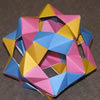### Plaited Origami Polyhedra

##### Age 7 to 16 Challenge Level:

These models have appeared around the Centre for Mathematical Sciences. Perhaps you would like to try to make some similar models of your own.### Triangles to Tetrahedra

##### Age 11 to 14 Challenge Level:

Imagine you have an unlimited number of four types of triangle. How many different tetrahedra can you make?### Tetrahedra Tester

##### Age 11 to 14 Challenge Level:

An irregular tetrahedron is composed of four different triangles. Can such a tetrahedron be constructed where the side lengths are 4, 5, 6, 7, 8 and 9 units of length?### Classifying Solids Using Angle Deficiency

##### Age 11 to 16 Challenge Level:

Toni Beardon has chosen this article introducing a rich area for practical exploration and discovery in 3D geometry### Icosian Game

##### Age 11 to 14 Challenge Level:

This problem is about investigating whether it is possible to start at one vertex of a platonic solid and visit every other vertex once only returning to the vertex you started at.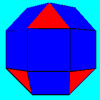### Rhombicubocts

##### Age 11 to 14 Challenge Level:

Each of these solids is made up with 3 squares and a triangle around each vertex. Each has a total of 18 square faces and 8 faces that are equilateral triangles. How many faces, edges and vertices. . . .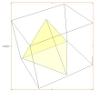### A Mean Tetrahedron

##### Age 11 to 14 Challenge Level:

Can you number the vertices, edges and faces of a tetrahedron so that the number on each edge is the mean of the numbers on the adjacent vertices and the mean of the numbers on the adjacent faces?### Which Solids Can We Make?

##### Age 11 to 14 Challenge Level:

Interior angles can help us to work out which polygons will tessellate. Can we use similar ideas to predict which polygons combine to create semi-regular solids?